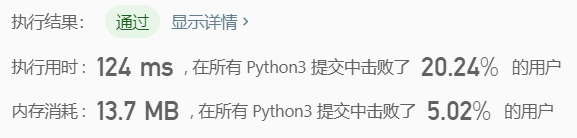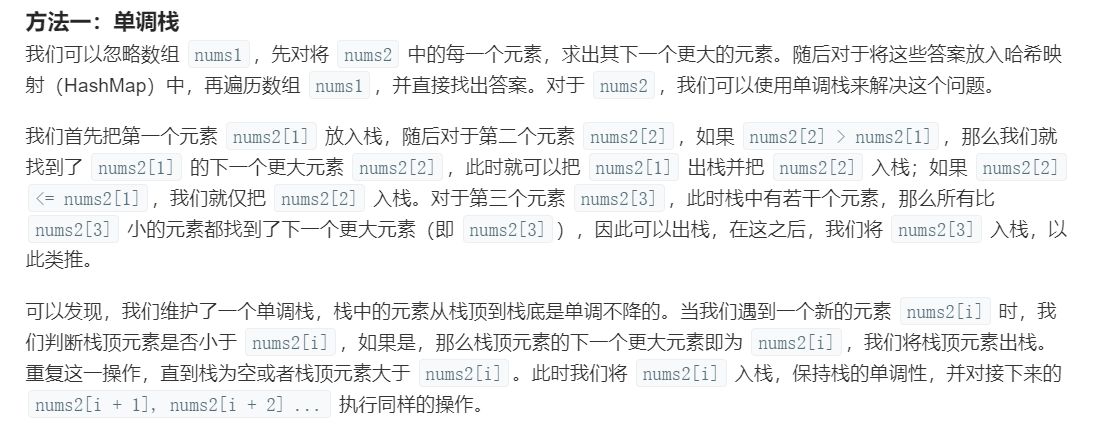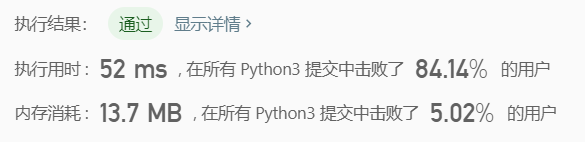# LeetCodeEasy-【496. 下一个更大元素 I】

nums1 中数字 x 的下一个更大元素是指 x 在 nums2 中对应位置的右边的第一个比 x 大的元素。如果不存在，对应位置输出-1。

对于num1中的数字4，你无法在第二个数组中找到下一个更大的数字，因此输出 -1。
对于num1中的数字1，第二个数组中数字1右边的下一个较大数字是 3。
对于num1中的数字2，第二个数组中没有下一个更大的数字，因此输出 -1。

对于num1中的数字2，第二个数组中的下一个较大数字是3。
对于num1中的数字4，第二个数组中没有下一个更大的数字，因此输出 -1。

nums1和nums2中所有元素是唯一的。
nums1和nums2 的数组大小都不超过1000。class Solution:
def nextGreaterElement(self, nums1: List[int], nums2: List[int]) -> List[int]:
ans = []
length = len(nums2)
for n1 in nums1:
if n1 not in nums2:
ans.append(-1)
else:
t = nums2.index(n1)
f = 0
for i in range(t + 1, length):
if nums2[i] > n1:
ans.append(nums2[i])
f = 1
break
if f == 0:
ans.append(-1)
return ansclass Solution:
def nextGreaterElement(self, nums1: List[int], nums2: List[int]) -> List[int]:
ans = []
stack = []
dict1 = {}
for n in nums2:
if stack !=[] and n > stack[-1]:
while stack != [] and n > stack[-1]:
dict1[stack[-1]] = n
stack.pop()
stack.append(n)

for n in nums1:
if n in dict1:
ans.append(dict1[n])
else:
ans.append(-1)
return ans©️2019 CSDN 皮肤主题: 书香水墨 设计师: CSDN官方博客